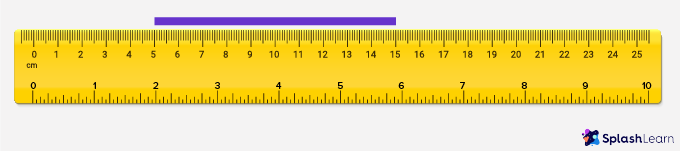# Ruler – Definition, Types, Examples, Practice Problems, FAQs

Home » Math-Vocabluary » Ruler – Definition, Types, Examples, Practice Problems, FAQs

## What is a Ruler?

A ruler can be defined as a tool or device used to measure length and draw straight lines.

A ruler is used to measure the length in both metric and customary units. The rulers are marked with standard distance in centimeters in the top and inches in the bottom and the intervals in the ruler are called hash marks.

## Types of Rulers:

There are different types of ruler depending on the material it is made of. Rulers are made in many different materials like wood, plastics, and metal.

Depending on the size of the ruler there are two types of ruler – a short ruler and a long ruler. Short rulers are 15 centimeters which are very convenient to carry and long rulers are 30 centimeters or 12 inches long, it is useful to draw larger drawings.

Depending on its usability, specially in the industries, rulers are also used in different formats like: Folding Ruler or Extension Ruler, Self-retracting Metal Tape, Plastic tape or a Soft Ruler.

Measuring Objects using a Ruler:

To measure the length of an object, place the zero hash mark of the ruler exactly along one end of the object. Align the object you are measuring along the edge of the ruler. Note the hashmark on the ruler along which the other side of the object ends.

Here, the shoelace is 17.6 cm long in metric units of length, and 7 inches long in customary units of length.

A centimeter is smaller than an inch. The long hash marks under which numbers are written on the ruler denote centimeters.

A millimeter is even smaller than a centimeter. The smaller hash marks between each centimeter represent millimeters.

10-millimeter hash marks make 1 centimeter.

An inch is bigger than a centimeter. The big long hash marks above which numbers are written on the opposite side of the centimeter ruler denote inches

How to read when the object is not placed at 0?

When the object is placed in the middle of the ruler and it doesn’t start at zero we need to observe the starting position where the object is placed.

Look at the example. Here a red stick is placed on the ruler.

The given object is not 12 cm long as it didn’t start at the edge of the ruler (zero). It started at 3. So start counting from 3 till 12. So the length of the object is 9 cm.

Mathematically we can calculate it as 12 – 3 = 9  cm.

How to draw using a ruler

• Place the ruler in a specific place where you want to draw a straight line.
• Take a ruler and mark a point at one end the other point at the given length
• Join the two points.

Uses of Ruler

• To measure the length and width of different objects.
• To draw straight lines.
• For engineering purposes where accurate measurements are required.
• For carpentry work.
• For taking measurements before and during construction.

## Solved Examples on Ruler

Example 1: Measure the length of the pencil in cm.

Solution:

The tip of the pencil is on zero (‘0’) and the end of the pencil is on hash showing 11. So, the length of the pencil is 11 cm.

Example 2: Measure the length of comb in inches.

Solution:

Zero hash mark is at one end and another end is at hash mark with 4 written on it. So the length of the comb is 4 inches.

Example 3: Write the reading shown by the pointer.

Solution:

The pointer is on the hash mark which is between 17 and 18. The pointer is on the 5th hash mark from 17. So, the pointer is on 17.5 cm

Example 4: Write the reading shown by the pointer.

Solution:

The pointer is on the side of the ruler that shows the measurement in inches. The pointer is on the hash that shows 5 written on it. So, the pointer is on 5 inch.

## Practice Problems on Ruler

1

### Elias wants to draw a line of given measurement. Which measuring instrument should he use to measure it?

Protractor
Compass
Divider
Ruler
CorrectIncorrect
Ruler is the standard tool for measuring distance.
2

### Find the measurement of the bar.5 cm
10 cm
15 cm
20 cm
CorrectIncorrect
The bar one end is at 5 cm and another end is at 15 cm.
The bar length = (15 – 5) cm = 10 cm
3

### Nathan has a plastic ruler scale in his compass box. Which of the following he won’t be able to measure using his scale?

Length of banana
Length of crayon
Length of book
Length of desk
CorrectIncorrect
Correct answer is: Length of banana
Bananas have a curved shape and plastic ruler is not flexible to measure the curve shape.
4

1 Millimeter
1 Centimeter
1 Inches
1 Feet
CorrectIncorrect